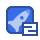# 数学建模-回归分析29收藏

22.1最小乘法 用最小二乘法佔计Bn,B1的值,即取B0,B的一组估计值B,B,使y2与 =B+Bx的误差平方和达到最小。若记 Q,A)=∑(y-B-Ax 贝 2(Bo,B1)=min Q(B,B)-2(,-Bo-B.,) 显然Q(0,B1)≥0,且关于B,月可微,则由多元函数存在极值的必要条件得 ∑(V-B-Bx) O 0B1 ∑x(y;--月x)=0 整理后,得到下面的方程组 nB+B∑x=∑y 4) A∑x+B∑x=∑ 此方稈组称为正规方程组,求解可以得到 (x1-x)(y-y) ∑(x-x) (5) B=-B1 称B0,B1为B0,B1的最小二乘估计,其中,x,分别是x与y2的样本均值,即 =∑x,j=∑ 关于B的计算公式还有一个更直观的表示方法,即 x-x)(v-y B1 ∑( y ∑(x-x)(y-y) S x 式中s=m12x-对),5=n2(,-,m是x与y的崖态相关系数 显然,当x,y都是标准化数据时,则有x=0,=0,S=1,S,=1。所以, 有 β0=0,B 回归方程为 由上可知,对标准化数据,B可以表示y与x的相关程度。 222B,B1的性质 作为一个随机变量,1有以下性质 B1是y的线性组合,它可以写成 B=∑k (6) 式中,k是固定的常量,k,= (x,-x 证明事实上 ∑(x-x)(-y)∑(x-)-元2(x-x) B1 ∑( 由于 (x1-x)=(n-n)=0 所以 y 2.因为β是随机变量y(i=1,2,…,n)的线性组合,而y是相互独立、且服从止 态分布的,所以,的抽样分布也服从正态分布。 3.点估计量B1是总体参数B的无偏估计,有 E(A)=E∑k1=∑E(y) ∑kE(月+Bx)=B∑k+B∑kx1 由于 ∑k,=∑ 0 ∑(x ∑(x,-x)(x1-x ∑kx=∑,-x x) 所以 E(B1)=B1 4.估计量B1的方差为 Var(Bu) (7) ∑( 这是因为 (A)=Vkx,=2Va()=22=口的 由于 ∑k2=∑ X -x ∑(x-x) 因此,式(7)得证。 5.对于总体模型中的参数β1,在它的所有线性无偏估计量中,最小二乘估计量B 具有最小的方差。 记任意个线性估计量 Ciyi 式中G是任意常数,c不全为零,i=1,2,…,n。要求B是B1的无偏估计量,即 E()=∑cE()=B 另一方面,由于E(y)=B0+B1x,所以又可以写成 E()=∑c(月+Rx)=∑c+月∑cx 为保证无偏性,c,要满足下列限制 0 Cixi 0 定义G1=k1+d1,其中k是式(6)中的组合系数,d是任意常数,则 Va(A)=2c=|22+24+2∑k 由于 ∑kd=∑k(-k)=∑c,--∑k2 ∑(x,-x) 0 ∑(x-x)2 i=1 ∑(x-x)2∑(x-x) 而 ∑k2 ∑(x-x) 所以 Var(B1)=var(B)+o2>d; ∑a2的最小值为零,所以,当∑2=0时,月1的方差最小但是,只有当d,=0 时,即c≡k时,才有∑d2=0。所以,最小乘估计量A在所有无偏估计量中具 有最小的方差。 同理,可以得出相应」点估计量β的统计性质。对」一元线性正态误差回归模型 来说,最小二乘估计量房是y1的线性组合,所以,它的抽样分布也是止态的。它是总 体参数B的去偏估让最,即 E(o)=Pu 同样可以证明 er(60) (8) 且β是B的线性无偏的最小方差估计量 22.3其它性质 用最小二乘法拟合的回归方程还有一些值待注意的性质: 1.残差和为 残差 e1=y1-y1,l 由第一个正规方程,得 ∑e=∑(-A0-月x1 2.拟合值立的平均值等干观测值的平均值,即 S_IS ;=y 按照第一正规方程,有 ∑(-B,-月x)=0 所以 ∑n=∑(+月x)=∑ 3.当第i次试验的残差以相应的自变量取值为杖重时,其加权残差和为零,即 x; ei (11) 这个结论由第个正规方程∑x(y--月x)=0即可得出 4.当第i次试验的残差以相应的因变量的拟合值为权重时,其加权残差和为岺, ∑e=0 (12) 这是因为 ∑(B+x)k=B∑c+B∑x 5.最小二乘回归线总是通过观测数的重心(x卫的 事实上,当自变量取值为τ时,由式(5) 0=-B1 所以 y=B+B Br)+x=y 2.3拟合效果分析 当根据一组观测数据得到最小二乘拟合方程后,必须考察一下,是否真的能由所得 的模型(,=B+Bx1)来较好地拟合观测值y,?用y=B0+B1x,能否较好地反映 (或者说解释)y值的取值变化?回归方程的质量如何?误差多大?对这些,都必须 予以正确的评估和分析。 23.1残差的样本方差 记残 i=1. 残差的样木均值为 ∑(y2-)=0 残差的样本方差为 MSE 由于有∑=0和∑xe=0的约束,所以,残差平方和有(n-2)个自由度。可 以证明,在对∑e2除以其自由度(n-2)后得到的MSE,是总体回归模型中 a2=r()的无偏估计量。记 S=√MSE=1,∑ (13) 一个好的拟合方程,其残差总和应越小越好。残差越小,拟合值与观测值越接近, 各观测点在拟合直线片围聚集的紧密程度越高,也就是说,拟合方程y=+月1x解释 y的能力越强。 另外,当S越小时,还说明残差值e的变异程度越小。由于残差的样本均值为零, 所以,其离散范围越小,拟合的模型就越为精确。 232判定系数(拟合优度) 对应于不同的x值,观测值y2的取值是不同的。建立一元线性回归模犁的日的, 就是试图以x的线性函数(B0+6x)来解释y的变异。那么,回归模犁j=B0+B1x 究竟能以多大的精度来解释y的变异呢?又有多大部分是无法用这个回归方程来解释 的呢 v1,y2…,yn的变异程度可用栏方差来测度,即 根据式(10),拟合值,2,…,的均值也是y,其变异程度可以用下式测度 下面看下s2与2之间的关系,有 ∑(-y)2=∑(1-j)2+∑(-y)2+2∑(1-)- 由于 ∑(y2-)-列=∑(y-月-Bx)0+A1x2-D) A∑(,-A0-Ax1)+B∑x(0-B0-Bx)-元∑(1-几一B1x)=0 因此,得到正交分解式为 ∑(-y)2+∑ (14 ST=∑(-y)2,这是原始数据y的总变异平方租,其自由度为d1=n-1 i=l ssR= 分,-),这是用拟合直线=+x可解释的变异平方租,其自 由度为df=1 SE=∑(y-),这是残差平方租,其的自由度为d=n-2 所以,有 SST'=SSR+SSE, dr=dp+dfp 从上式可以看出,y的变异是由两方面的原因引起的:一是由于x的取值不同,而 给y带米的系统性变异;另一个是由除x以外的其它因素的影响 注意到对于一个确定的样本(一组实现的观测值),SST是一个定值。所以,可解 释变异SSR越大,则必然有残差SSE越小。这个分解式可同时丛两个方面说明拟合方 程的优良程度 1SR越太,用回归方程来解上变异的部分越太,回归方程对原数据解释得 (2)SSE越小,观测值;绕回归直线葳紧窣,回归方程对原数据的拟合效果載好。 因此,可以定义一个测量标准来说明回归方程对原始数据的拟合程度,这就是所谓 的判定系数,有些文献上也称之为拟合优度 判定系数是指可解释的变异占总变异的百分比,用R表示,有 SSR SSE R (15) SST SST 从判定系数的定义看,R2有以下简单性质: (1)0≤R2≤1; (2)当R2=1时,有SSR=SST,也就是说,此时原数据的总变异完仝可以由拟 合值的变异来解释,并且残差为零(SSE=0),即拟合点与原数据完全吻合; 3)当R2=0时,回归方程完全不能解释原数据的总变异,y的变异完全由与x 无关的因素引起,这时SSE=SST。 测定系数时一个很有趣的指标:一方面它可以从数据变异的角度指出可解释的变异 占总变异的白分比,从而说明回归直线拟合的优良程度;另一方面,它还可以从相关性 的角度,说明原因变量y与拟合变量j的相关程度,从这个角度看,拟合变量j与原 变量y的相关度越人,拟合直线的优良度就越高。 看下面的式子 SSR ∑(,-2∑(+e-- R SST ∑(y2-y)2∑(y2-元)2∑(-)2 在推导中,注意有 ∑e(-列=∑e-∑e=0 所以,R2又等上y与合变量上的相关系数平方。 还可以证明,√R2等王与自变量x的相关系数,而相关系数的正、负号与回归 系数β的符号相同。 24显著性检验 241回归模型的线性关系检验 在拟合回归方程之前,我们曾假设数据总体是符合线性正态误差模型的,也就是说, y与x之间的关系是线性关系,即 y1=B0+Bx1+E1,E1~N(0,a2),i=1,2,…,n 然而,这种假设是否真实,还需进行检验 对」一个实际观测的样木,虽然可以用判定系数R2说明y与j的相关程度,但是, 样本测度指标具有一定的随机因素,还不足以肯定y与x的线性关系 假设y与x之间存在线性关系,则总体模型为 β+B1x+E1,i=1,2, 如果B1≠0,则称这个模型为全模型 用最小二乘法拟合仝模型,并求出误差平方租为 SE=∑(y-) 现给出假设H:B1=0。如果H假设成立,则 y1=B0+E1 这个模型被称为选模型。用最小二乘法拟合这个模型,则有 B0=-B07= 因此,对所有的i=1,2,…,n,有

...展开详情

• 2
资源
• 0
粉丝
•等级数学建模-回归分析 10积分/C币 立即下载
1/2610积分/C币 立即下载